# modelAccuracy

Compute R-square, RMSE, correlation, and sample mean error of predicted and observed EADs

## Syntax

``AccMeasure = modelAccuracy(eadModel,data)``
``[AccMeasure,AccData] = modelAccuracy(___,Name,Value)``

## Description

example

````AccMeasure = modelAccuracy(eadModel,data)` computes the R-square, root mean square error (RMSE), correlation, and sample mean error of observed vs. predicted exposure at default (EAD) data. `modelAccuracy` supports comparison against a reference model and also supports different correlation types. By default, `modelAccuracy` computes the metrics in the EAD scale. You can use the `ModelLevel` name-value pair argument to compute metrics using the underlying model's transformed scale.```

example

````[AccMeasure,AccData] = modelAccuracy(___,Name,Value)` specifies options using one or more name-value pair arguments in addition to the input arguments in the previous syntax.```

## Examples

collapse all

This example shows how to use `fitEADModel` to create a `Tobit` model and then use `modelAccuracy` to compute the R-Square, RMSE, correlation, and sample mean error of predicted and observed EAD.

```load EADData.mat head(EADData)```
```ans=8×6 table UtilizationRate Age Marriage Limit Drawn EAD _______________ ___ ___________ __________ __________ __________ 0.24359 25 not married 44776 10907 44740 0.96946 44 not married 2.1405e+05 2.0751e+05 40678 0 40 married 1.6581e+05 0 1.6567e+05 0.53242 38 not married 1.7375e+05 92506 1593.5 0.2583 30 not married 26258 6782.5 54.175 0.17039 54 married 1.7357e+05 29575 576.69 0.18586 27 not married 19590 3641 998.49 0.85372 42 not married 2.0712e+05 1.7682e+05 1.6454e+05 ```
```rng('default'); NumObs = height(EADData); c = cvpartition(NumObs,'HoldOut',0.4); TrainingInd = training(c); TestInd = test(c);```

Select Model Type

Select a model type for `Tobit` or `Regression`.

`ModelType =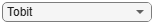"Tobit";`

Select Conversion Measure

Select a conversion measure for the EAD response values.

`ConversionMeasure =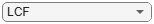"LCF";`

Create `Tobit` EAD Model

Use `fitEADModel` to create a `Tobit` model using `EADData`.

```eadModel = fitEADModel(EADData,ModelType,'PredictorVars',{'UtilizationRate','Age','Marriage'}, ... 'ConversionMeasure',ConversionMeasure,'DrawnVar','Drawn','LimitVar','Limit','ResponseVar','EAD'); disp(eadModel);```
``` Tobit with properties: CensoringSide: "both" LeftLimit: 0 RightLimit: 1 ModelID: "Tobit" Description: "" UnderlyingModel: [1x1 risk.internal.credit.TobitModel] PredictorVars: ["UtilizationRate" "Age" "Marriage"] ResponseVar: "EAD" LimitVar: "Limit" DrawnVar: "Drawn" ConversionMeasure: "lcf" ```

Display the underlying model. The underlying model's response variable is the transformation of the EAD response data. Use the `'LimitVar'` and `'DrwanVar'` name-value arguments to modify the transformation.

`disp(eadModel.UnderlyingModel);`
```Tobit regression model: EAD_lcf = max(0,min(Y*,1)) Y* ~ 1 + UtilizationRate + Age + Marriage Estimated coefficients: Estimate SE tStat pValue __________ __________ ________ ________ (Intercept) 0.22735 0.025045 9.0776 0 UtilizationRate 0.47364 0.016536 28.643 0 Age -0.0013929 0.00061488 -2.2654 0.023537 Marriage_not married -0.006888 0.012113 -0.56863 0.56964 (Sigma) 0.36419 0.0038746 93.995 0 Number of observations: 4378 Number of left-censored observations: 0 Number of uncensored observations: 4377 Number of right-censored observations: 1 Log-likelihood: -1791.06 ```

EAD prediction operates on the underlying compact statistical model and then transforms the predicted values back to the EAD scale. You can specify the `predict` function with different options for the `'ModelLevel'` name-value argument.

```predictedEAD = predict(eadModel,EADData(TestInd,:),'ModelLevel','ead'); predictedConversion = predict(eadModel,EADData(TestInd,:),'ModelLevel','ConversionMeasure');```

For model validation, use `modelDiscrimination`, `modelDiscriminationPlot`, `modelAccuracy`, and `modelAccuracyPlot`.

Use `modelDiscrimination` and then `modelDiscriminationPlot` to plot the ROC curve.

```ModelLevel =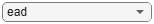"ead"; [DiscMeasure1,DiscData1] = modelDiscrimination(eadModel,EADData(TestInd,:),'ModelLevel',ModelLevel); modelDiscriminationPlot(eadModel,EADData(TestInd, :),'ModelLevel',ModelLevel,'SegmentBy','Marriage');```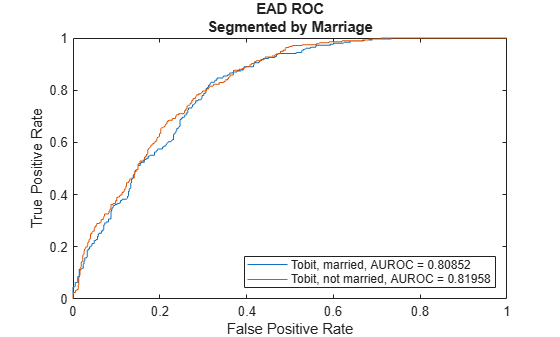Use `modelAccuracy` and then `modelAccuracyPlot` to show a scatter plot of the predictions.

```YData =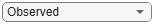"Observed"; [AccMeasure1,AccData1] = modelAccuracy(eadModel,EADData(TestInd,:),'ModelLevel',ModelLevel)```
```AccMeasure1=1×4 table RSquared RMSE Correlation SampleMeanError ________ _____ ___________ _______________ Tobit 0.39127 42545 0.62552 -1713.1 ```
```AccData1=1751×3 table Observed Predicted_Tobit Residuals_Tobit __________ _______________ _______________ 44740 15177 29563 54.175 8900.3 -8846.1 987.39 13430 -12443 9606.4 7422.4 2184 83.809 27852 -27768 73538 46229 27309 96.949 5582.8 -5485.9 873.21 4527.1 -3653.9 328.35 6079.8 -5751.5 55237 28295 26942 30359 19177 11182 39211 28753 10457 2.0885e+05 1.0725e+05 1.016e+05 1921.7 20132 -18210 15230 5526.4 9703.5 20063 9501.2 10562 ⋮ ```
`modelAccuracyPlot(eadModel,EADData(TestInd,:),'ModelLevel',ModelLevel,'YData',YData);`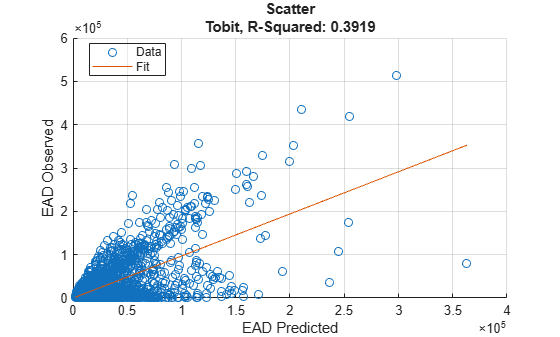## Input Arguments

collapse all

Loss given default model, specified as a previously created `Regression` or `Tobit` object using `fitEADModel`.

Data Types: `object`

Data, specified as a `NumRows`-by-`NumCols` table with predictor and response values. The variable names and data types must be consistent with the underlying model.

Data Types: `table`

### Name-Value Arguments

Specify optional comma-separated pairs of `Name,Value` arguments. `Name` is the argument name and `Value` is the corresponding value. `Name` must appear inside quotes. You can specify several name and value pair arguments in any order as `Name1,Value1,...,NameN,ValueN`.

Example: ```[AccMeasure,AccData] = modelAccuracy(eadModel,data(TestInd,:),'DataID','Testing','CorrelationType','spearman')```

Correlation type, specified as the comma-separated pair consisting of `'CorrelationType'` and a character vector or string.

Data Types: `char` | `string`

Data set identifier, specified as the comma-separated pair consisting of `'DataID'` and a character vector or string. The `DataID` is included in the output for reporting purposes.

Data Types: `char` | `string`

Model level, specified as the comma-separated pair consisting of `'ModelLevel'` and a character vector or string.

Note

`Regression` models support all three model levels, but a `Tobit` model supports model levels only for `'ead'` and `'conversionMeasure'`.

Data Types: `char` | `string`

EAD values predicted for `data` by the reference model, specified as the comma-separated pair consisting of `'ReferenceEAD'` and a `NumRows`-by-`1` numeric vector. The `modelAccuracy` output information is reported for both the `eadModel` object and the reference model.

Data Types: `double`

Identifier for the reference model, specified as the comma-separated pair consisting of `'ReferenceID'` and a character vector or string. `'ReferenceID'` is used in the `modelAccuracy` output for reporting purposes.

Data Types: `char` | `string`

## Output Arguments

collapse all

Accuracy measure, returned as a table with columns `'RSquared'`, `'RMSE'`, `'Correlation'`, and `'SampleMeanError'`. `AccMeasure` has one row if only the `eadModel` accuracy is measured and it has two rows if reference model information is given. The row names of `AccMeasure` report the model ID and data ID (if provided).

Accuracy data, returned as a table with observed EAD values, predicted EAD values, and residuals (observed minus predicted). Additional columns for predicted and residual values are included for the reference model, if provided. The `ModelID` and `ReferenceID` labels are appended in the column names.

collapse all

### Model Accuracy

Model accuracy measures the accuracy of the predicted probability of EAD values using different metrics.

• R-squared — To compute the R-squared metric, `modelAccuracy` fits a linear regression of the observed EAD values against the predicted EAD values:

`$EA{D}_{obs}=a+b\ast EA{D}_{pred}+\epsilon$`

The R-square of this regression is reported. For more information, see Coefficient of Determination (R-Squared).

• RMSE — To compute the root mean square error (RMSE), `modelAccuracy` uses the following formula where N is the number of observations:

`$RMSE=\sqrt{\frac{1}{N}{\sum }_{i=1}^{N}\left(EA{D}_{i}^{obs}-EA{D}_{i}^{pred}{\right)}^{2}}$`

• Correlation — This metric is the correlation between the observed and predicted EAD:

`$corr\left(EA{D}_{obs},EA{D}_{pred}\right)$`

For more information and details about the different correlation types, see `corr`.

• Sample mean error — This metric is the difference between the mean observed EAD and the mean predicted EAD or, equivalently, the mean of the residuals:

`$SampleMeanError=\frac{1}{N}{\sum }_{i=1}^{N}\left(EA{D}_{i}^{obs}-EA{D}_{i}^{pred}\right)$`

 Baesens, Bart, Daniel Roesch, and Harald Scheule. Credit Risk Analytics: Measurement Techniques, Applications, and Examples in SAS. Wiley, 2016.

 Bellini, Tiziano. IFRS 9 and CECL Credit Risk Modelling and Validation: A Practical Guide with Examples Worked in R and SAS. San Diego, CA: Elsevier, 2019.

 Brown, Iain. Developing Credit Risk Models Using SAS Enterprise Miner and SAS/STAT: Theory and Applications. SAS Institute, 2014.

 Roesch, Daniel and Harald Scheule. Deep Credit Risk. Independently published, 2020.